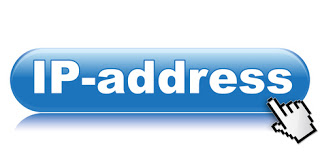# Free IP and Port For Anonymous and Fast BrowsingAndroid Users Just go to Settings and choose your network, then click AP, select the network APN , and finally Use any of these fast and free Proxy servers to see wonders on your Internet SpeedIP ADDRESS    ===  PORT  ====    COUNTRY

173.161.0.227 == 80 == US == United States
37.187.121.56 == 3128 == FR == France
47.88.104.219 == 80 == CA == Canada
178.32.153.219 == 80 == FR == France
191.53.51.22 == 3128 == BR == Brazil  == elite proxy
74.202.77.211 == 80 == US == United States== elite proxy
212.80.167.93 == 3128 == ES == Spain == elite proxy
203.223.143.51 == 8080 == MY == Malaysia
166.70.157.58 == 80 == US == United States == elite proxy
31.172.132.9 == 8080 == RU == Russian Federation
213.136.89.121 == 80 == DE == Germany
97.77.104.22 == 80 == US == United States
213.136.77.246 == 80 == DE == Germany
202.155.210.2 == 8080 == HK == Hong Kong == elite proxy
201.55.46.6 == 80 == BR == Brazil === elite proxy
200.68.27.100 == 3128 == CL == Chile
146.0.73.14 == 80 == NL == Netherlands
178.33.23.150 == 8080 == FR == France == elite proxy
186.229.28.138 == 80 == BR == Brazil == elite proxy
171.100.123.143 == 3128 == TH == Thailand
169.255.144.174 == 8000 == ZA == South Africa == transparent
41.170.1.242 == 8080 == ZA == South Africa == transparent
41.169.35.199 == 8080 == ZA == South Africa== transparent
41.144.126.221 == 8088 == ZA == South Africa == transparent
105.112.6.28 == 8080 == NG == Nigeria== transparent
197.210.252.40 == 8080 == NG == Nigeria ==  transparent
41.184.208.124 == 8080 == NG == Nigeria == transparent
41.212.73.2 == 3128 == KE == Kenya == transparent

This post first appeared on ViberTechs, please read the originial post: here

# Share the post

Free IP and Port For Anonymous and Fast Browsing

×